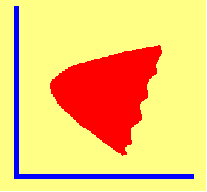4.1 OVERVIEW

You have learned that portfolio risk is measured by the standard deviation of portfolio return, while portfolio return is measured by the expected return. The aim of this chapter is to show you how different combinations of portfolio weights affect portfolio risk and return. By applying CAPM Tutor's Markowitz Diversification chapter, you can view dynamically the impact of changing portfolio weights upon the risk and return characteristics of a portfolio. This can be done using the default data set or the data set of your choice. Details on how to interact with the program can be found using FastStart.

Recall from Chapter 3, (see Overview), that the contribution of an individual security to portfolio risk depends upon both a security's own variance and its covariance of returns with all other securities in the portfolio. The covariance of a security's return with other securities obviously depends on what other securities are in the portfolio. Furthermore, a security's contributions to both the variance and covariance depend upon the weight of the security in the portfolio.

In an equally weighted portfolio, you have learned that the portfolio's standard deviation is dominated by covariance terms. That is, a security's variance contributes a source of risk that can be diversified away by forming a large enough portfolio. Large does not mean a portfolio has to consist of 10,000 securities. Even equally weighted portfolios with as few as 15 securities can provide significant gains in reducing risk.

The seminal contribution to portfolio theory by Markowitz (1952) addresses the impact of choosing portfolio weights optimally upon the diversification of risk. The portfolio selection technique introduced by this important work, is referred to as Markowitz diversification.

Markowitz diversification improves upon naive diversification. It takes advantage of the fact that risk can be reduced further by the management of portfolio weights. CAPM Tutor allows you to observe, for yourself, how changing portfolio weights affects both the risk and return of a portfolio.

The management of portfolio weights allows you to control risk in both small and large portfolios. In a large portfolio, you can control the contribution to total portfolio risk due to the covariance terms. In a small portfolio, you can manage the contribution from both variance and covariance terms. In this Chapter in topic 4.2, Three-Firm Case: combining securities into portfolios, you can develop your intuition by applying CAPM Tutor to the Three-Firm Case. For example, you can compare a naively diversified portfolio to portfolios with different weights of securities.

After you have an understanding of how changing portfolio weights affects the risk and return of a portfolio, we pose the question: What is the best portfolio for a target expected return?

The contribution of Markowitz transforms the general investment problem into a formal theory of portfolio selection. In this chapter, we guide you through this theory. In topic 4.3, Investment Opportunity Set, we look at a "scatter plot" of a large number of randomly selected portfolios in the Three-Firm Case. You can use CAPM Tutor to actually experiment with forming portfolios and produce a similar plot. What you will observe is that portfolios plotted in risk/return space will fall within a well-defined boundary. Calculation of this boundary plays a central role in the theory.

This topic also introduces the concept of dominating portfolios. Intuitively, we know that portfolio A dominates portfolio B if A is less risky than B and gives you the same expected return. All undominated portfolios lie on the boundary called the minimum-variance frontier. Despite its name, what is usually depicted is the standard deviation, not the variance, of portfolios, but we follow the common practice and refer to the boundary as the minimum-variance frontier.

To calculate the minimum-variance frontier, you need to learn about the mathematical properties that characterize minimum-variance portfolios. We develop these mathematical properties in three steps. Topic 4.5, Two-Firm Analysis introduces you to the general problem when there are only two securities. For each level of expected return, we look for the portfolio with the smallest standard deviation (or variance). The general case is covered in topic 4.6, titled N-Security Case which we then apply to the Three-Firm Case in topic 4.9.

If you like return and want to avoid risk, you would always pick portfolios corresponding to the minimum-variance frontier. Some portfolios are dominated by others; that is, they have the same variance but a higher expected return. This set of undominated portfolios is called the efficient frontier.

Remember that CAPM Tutor allows you to solve these problems for the two-firm, three-firm or general case at the click of a mouse. These support help topics permit you to see exactly what is happening when the mouse is clicked!

The final topic in this chapter, topic 4.10, The Shape of the Frontier, tells you why the frontier assumes a particular shape.

previous topic

next topic